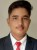# Calculators Created by Devendar KachhwahaIndian Institute of technology (IIT-BHU), Varanasi
23
Formulas Created
3
Formulas Verified
15
Across Categories

## List of Calculators by Devendar Kachhwaha

Following is a combined list of all the calculators that have been created and verified by Devendar Kachhwaha. Devendar Kachhwaha has created 23 and verified 3 calculators across 15 different categories till date.
Created Area of X Shape given Bottom or Top Angle
Created Area of X Shape given Left or Right Angle
Verified Arithmetic Mean of Four Numbers
5 More Arithmetic Mean Calculators
Created Bar Length of X Shape given Outer Arm Length
Created Bar Thickness of X Shape given Crossing Length and Bottom or Top Angle
Created Bar Thickness of X Shape given Perimeter and Arm Lengths
Created Bottom and Top Angle of X Shape given Crossing Length
Created Bottom and Top Angle of X Shape given Left or Right Angle
Created Nth Catalan Number
13 More Combinations Calculators
Created Crossing Length of X Shape given Bottom or Top Angle
Created Crossing Length of X Shape given Inner and Outer Arm Lengths
Created Crossing Length of X Shape given Left or Right Angle
Created Crossing Length of X Shape given Perimeter
Verified First Term of Arithmetic Progression
2 More First Term of Arithmetic Progression Calculators
Created Height of X Shape given Bottom or Top Angle
1 More Height of X Shape Calculators
Created Inner Arm Length of X Shape given Bottom or Top Angle
Created Inner Arm Length of X Shape given Crossing Length
Created Inner Arm Length of X Shape given Perimeter
1 More Inner Arm Length of X Shape Calculators
Created Left and Right Angle of X Shape given Bottom or Top Angle
Verified Nth Term of Arithmetic Progression given Sum of First N Terms
5 More Nth Term of Arithmetic Progression Calculators
Created Outer Arm Length of X Shape
Created Outer Arm Length of X Shape given Crossing and Inner Arm Length
Created Outer Arm Length of X Shape given Perimeter and Inner Arm Length
Created Perimeter of X Shape given Arm Lengths
Created Perimeter of X Shape given Crossing Length
Created Width of X Shape given Inner Arm Length and Bottom or Top AngleLet Others Know